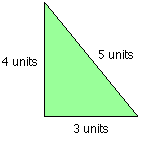#### You may also like### Square Pegs

Which is a better fit, a square peg in a round hole or a round peg in a square hole?### The Old Goats

A rectangular field has two posts with a ring on top of each post. There are two quarrelsome goats and plenty of ropes which you can tie to their collars. How can you secure them so they can't fight each other but can reach every corner of the field?### Isosceles

Prove that a triangle with sides of length 5, 5 and 6 has the same area as a triangle with sides of length 5, 5 and 8. Find other pairs of non-congruent isosceles triangles which have equal areas.

# Pythagorean Triples

##### Age 11 to 14 Challenge Level:

The smallest right-angled triangle where the lengths of all the sides are integers, has sides of length $3$, $4$ and $5$ units. This triangle has an area of $6$ square units and a perimeter of $12$ units:• How many right-angled triangles are there with sides that are all integers less than $100$ units?
• In how many of these triangles is the area one half of the perimeter?